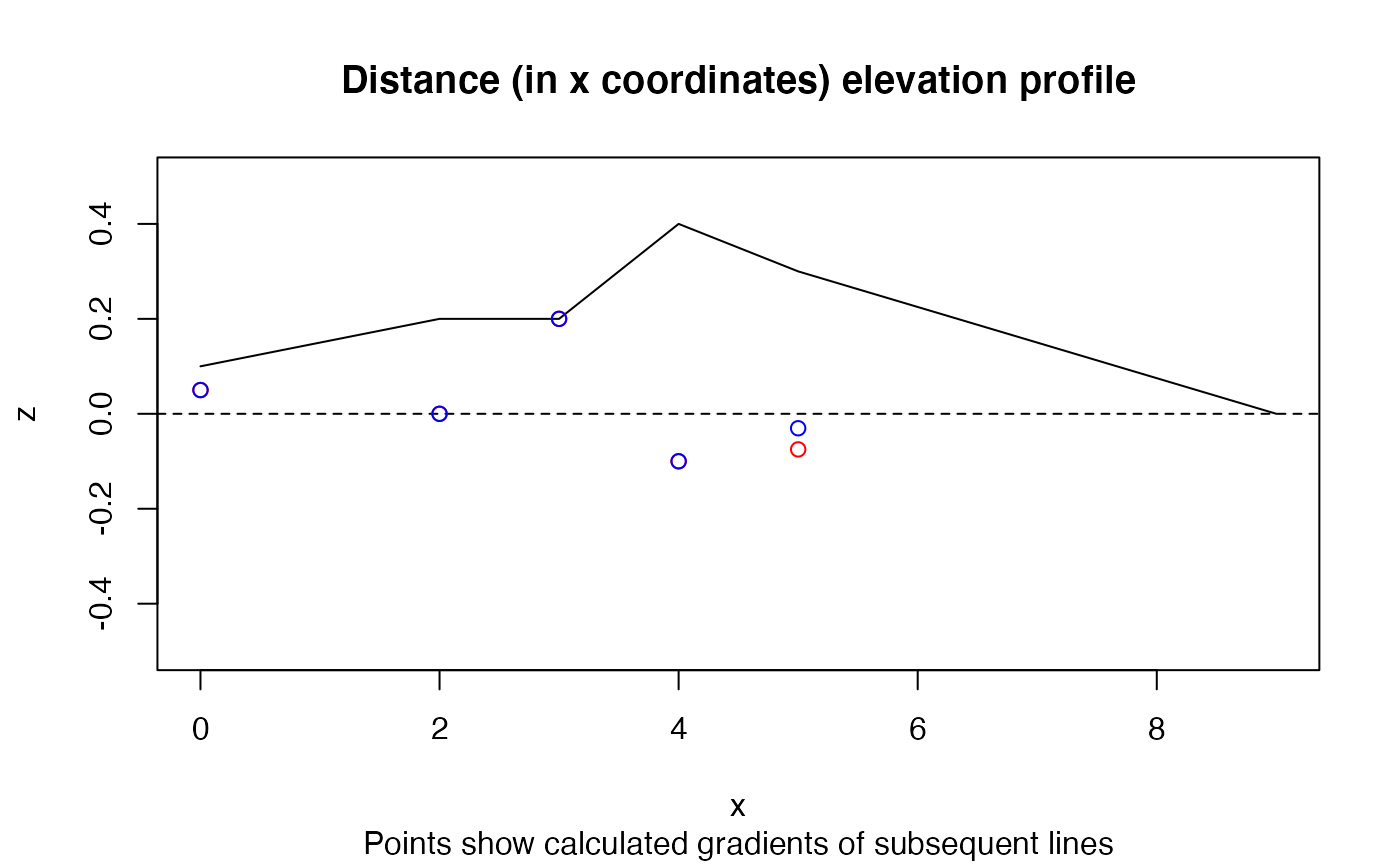Calculate the gradient of line segments from a 3D matrix of coordinates

slope_matrix(m, elevations = m[, 3], lonlat = TRUE)

slope_matrix_mean(m, elevations = m[, 3], lonlat = TRUE, directed = FALSE)

slope_matrix_weighted(m, elevations = m[, 3], lonlat = TRUE, directed = FALSE)

## Arguments

m Matrix containing coordinates and elevations. The matrix should have three columns: x, y, and z, in that order. Typically these correspond to location in the West-East, South-North, and vertical elevation axes respectively. In data with geographic coordinates, Z values are assumed to be in metres. In data with projected coordinates, Z values are assumed to have the same units as the X and Y coordinates. Elevations in same units as x (assumed to be metres). Default value: m[, 3], meaning the 'z' coordinate in a matrix of coordinates. Are the coordinates in lon/lat (geographic) coordinates? TRUE by default. Should the value be directed? FALSE by default. If TRUE the result will be negative when it represents a downslope (when the end point is lower than the start point).

## Value

A vector of slope gradients associated with each linear element (each line between consecutive vertices) associated with linear features. Returned values for slope_matrix_mean() and slope_matrix_weighted() are summary statistics for all linear elements in the linestring. The output value is a proportion representing the change in elevation for a given change in horizontal movement along the linestring. 0.02, for example, represents a low gradient of 2% while 0.08 represents a steep gradient of 8%.

## Examples

x = c(0, 2, 3, 4, 5, 9)
y = c(0, 0, 0, 0, 0, 9)
z = c(1, 2, 2, 4, 3, 0) / 10
m = cbind(x, y, z)
slope_matrix_weighted(m, lonlat = FALSE)
#>  0.04714167slope_matrix_weighted(m, lonlat = FALSE, directed = TRUE)
#>  -0.04714167# 0 value returned if no change in elevation:
slope_matrix_weighted(m,lonlat = FALSE, directed = TRUE,
elevations = c(1, 2, 2, 4, 3, 1))
#>  0slope_matrix_mean(m, lonlat = FALSE)
#>  0.07609208slope_matrix_mean(m, lonlat = FALSE, directed = TRUE)
#>  -0.07609208plot(x, z, ylim = c(-0.5, 0.5), type = "l")
(gx = slope_vector(x, z))
#>   0.050  0.000  0.200 -0.100 -0.075(gxy = slope_matrix(m, lonlat = FALSE))
#>   0.05000000  0.00000000  0.20000000 -0.10000000 -0.03046038abline(h = 0, lty = 2)
points(x[-length(x)], gx, col = "red")
points(x[-length(x)], gxy, col = "blue")
title("Distance (in x coordinates) elevation profile",
sub = "Points show calculated gradients of subsequent lines")